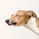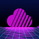30506 views
Beta divergence indicator, you can exchange the Price Percentage Oscillator ( PPO ) for any oscillator. You can play around with the look-back period if you want to use long term divergences.
WARNING:
Because its impossible to make the look-back period of the lowest/highest functions dynamic, the indicator can plot divergences sometimes at places where there are none, however in my experience this does not occur so much. Also it is impossible to plot isolated lines, so its hard to visually see which divergence(s) exactly is(are) being signaled. Once these features become possible I can really refine the indicator.
Open-source script

In true TradingView spirit, the author of this script has published it open-source, so traders can understand and verify it. Cheers to the author! You may use it for free, but reuse of this code in a publication is governed by House Rules. You can favorite it to use it on a chart.

Want to use this script on a chart?
```study(title = "Pekipek's PPO Divergence BETA", shorttitle = "PP's_PPO_DIV")
source = close
long_term_div = input(true, title="Use long term Divergences?")
div_lookback_period = input(55, minval=1, title="Lookback Period")
fastLength = input(12, minval=1), slowLength=input(26,minval=1)
signalLength=input(9,minval=1)
smoother = input(2,minval=1)
fastMA = ema(source, fastLength)
slowMA = ema(source, slowLength)
macd = fastMA - slowMA
macd2=(macd/slowMA)*100
d = sma(macd2, smoother) // smoothing PPO

bullishPrice = low

priceMins = bullishPrice > bullishPrice and bullishPrice < bullishPrice or
low == low and low < low and low < low or
low == low and low == low and low < low and low < low or
low == low and low == low and low and low == low and low < low and low < low // this line identifies bottoms and plateaus in the price
oscMins= d > d and d < d // this line identifies bottoms in the PPO

BottomPointsInPPO = oscMins

bearishPrice = high
priceMax = bearishPrice < bearishPrice and bearishPrice > bearishPrice or
high == high and high > high and high > high or
high == high and high == high and high > high and high > high or
high == high and high == high and high and high == high and high > high and high > high  // this line identifies tops in the price
oscMax = d < d and d > d   // this line identifies tops in the PPO

TopPointsInPPO = oscMax

currenttrough4=valuewhen (oscMins, d, 0) // identifies the value of PPO at the most recent BOTTOM in the PPO
lasttrough4=valuewhen (oscMins, d, 1) // NOT USED identifies the value of PPO at the second most recent BOTTOM in the PPO
currenttrough5=valuewhen (oscMax, d, 0) // identifies the value of PPO at the most recent TOP in the PPO
lasttrough5=valuewhen (oscMax, d, 1) // NOT USED identifies the value of PPO at the second most recent TOP in the PPO

currenttrough6=valuewhen (priceMins, low, 0) // this line identifies the low (price) at the most recent bottom in the Price
lasttrough6=valuewhen (priceMins, low, 1) // NOT USED this line identifies the low (price) at the second most recent bottom in the Price
currenttrough7=valuewhen (priceMax, high, 0) // this line identifies the high (price) at the most recent top in the Price
lasttrough7=valuewhen (priceMax, high, 1) // NOT USED this line identifies the high (price) at the second most recent top in the Price

delayedlow = priceMins and barssince(oscMins) < 3 ? low : na
delayedhigh = priceMax and barssince(oscMax) < 3 ? high : na

// only take tops/bottoms in price when tops/bottoms are less than 5 bars away
filter = barssince(priceMins) < 5 ? lowest(currenttrough6, 4) : na
filter2 = barssince(priceMax) < 5 ? highest(currenttrough7, 4) : na

//delayedbottom/top when oscillator bottom/top is earlier than price bottom/top
y11 = valuewhen(oscMins, delayedlow, 0)
y12 = valuewhen(oscMax, delayedhigh, 0)

// only take tops/bottoms in price when tops/bottoms are less than 5 bars away, since 2nd most recent top/bottom in osc
y2=valuewhen(oscMax, filter2, 1) // identifies the highest high in the tops of price with 5 bar lookback period SINCE the SECOND most recent top in PPO
y6=valuewhen(oscMins, filter, 1) // identifies the lowest low in the bottoms of price with 5 bar lookback period SINCE the SECOND most recent bottom in PPO

long_term_bull_filt = valuewhen(priceMins, lowest(div_lookback_period), 1)
long_term_bear_filt = valuewhen(priceMax, highest(div_lookback_period), 1)

y3=valuewhen(oscMax, currenttrough5, 0) // identifies the value of PPO in the most recent top of PPO
y4=valuewhen(oscMax, currenttrough5, 1) // identifies the value of PPO in the second most recent top of PPO

y7=valuewhen(oscMins, currenttrough4, 0) // identifies the value of PPO in the most recent bottom of PPO
y8=valuewhen(oscMins, currenttrough4, 1) // identifies the value of PPO in the SECOND most recent bottom of PPO

y9=valuewhen(oscMins, currenttrough6, 0)
y10=valuewhen(oscMax, currenttrough7, 0)

bulldiv= BottomPointsInPPO ? d : na // plots dots at bottoms in the PPO
beardiv= TopPointsInPPO ? d: na // plots dots at tops in the PPO

i = currenttrough5 < highest(d, div_lookback_period) // long term bearish oscilator divergence
i2 = y10 > long_term_bear_filt // long term bearish top divergence
i3 = delayedhigh > long_term_bear_filt // long term bearish delayedhigh divergence

i4 = currenttrough4 > lowest(d, div_lookback_period) // long term bullish osc divergence
i5 = y9 < long_term_bull_filt // long term bullish bottom div
i6 = delayedlow < long_term_bull_filt // long term bullish delayedbottom div

plot(d, color=white)
plot(bulldiv, title = "Tops", color=aqua, style=circles, linewidth=4, offset= -1)
plot(beardiv, title = "Bottoms", color=red, style=circles, linewidth=4, offset= -1)

plot(y10>y2 and oscMax and y3 < y4 ? d :na, title = "Bearish Divergence2", color=orange, style= circles, linewidth=4)
plot(y9<y6 and oscMins and y7 > y8 ? d :na, title = "Bullish Divergence2", color=purple, style=circles, linewidth=4)
plot(delayedlow<y6 and y7 > y8 ? d :na, title = "Bullish Divergence2", color=purple, style=circles, linewidth=4)
plot(delayedhigh>y2 and y3 < y4 ? d :na, title = "Bearish Divergence2", color=orange, style= circles, linewidth=4)

plot(long_term_div and oscMax and i and i2 ? d :na, title = "Bearish Divergence2", color=orange, style= circles, linewidth=4)
plot(long_term_div and oscMins and i4 and i5 ? d : na, title = "Bullish Divergence2", color=purple, style=circles, linewidth=4)
plot(long_term_div and i and i3 ? d :na, title = "Bearish Divergence2", color=orange, style= circles, linewidth=4)
plot(long_term_div and i4 and i6 ? d : na, title = "Bullish Divergence2", color=purple, style=circles, linewidth=4)
```